# 《数据结构与算法》第6章 两个栈实现队列 (C语言)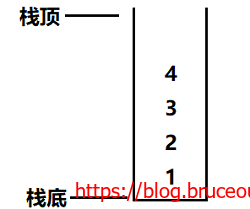==队列的特点==是先进先出，例如，把序列1,2,3,4,存入队列中。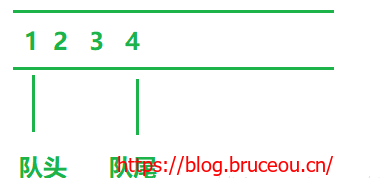## 6.1入队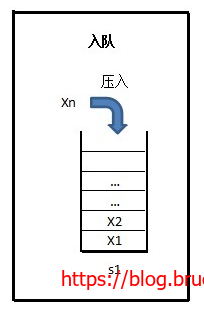int Sequeue_En(seqstack_t *stack1,int k)
{
if(stack1 == NULL)
{
return -1;
}

PushStack(stack1, k);

return 0;
}

## 6.2出队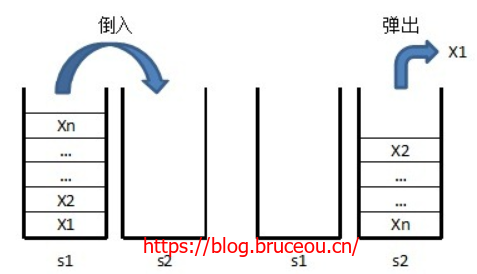data_t Dequeue_De(seqstack_t *stack1,seqstack_t *stack2,int *x)
{
if(stack1 == NULL || stack2 == NULL)
{
return -1;
}
else
{
if(EmptyStack(stack1) && EmptyStack(stack2))
{
printf("This queue is empty\n");
}
if(EmptyStack(stack2) == 0)
{
PopStack(stack2,x);
}
else
{
while(EmptyStack(stack1) == 0)
{
PopStack(stack1,x);
if(FullStack(stack2))
{
printf("stack full!\n");
}
else
{
PushStack(stack2,*x);
}
}
PopStack(stack2,x);
}
}

return 0;
}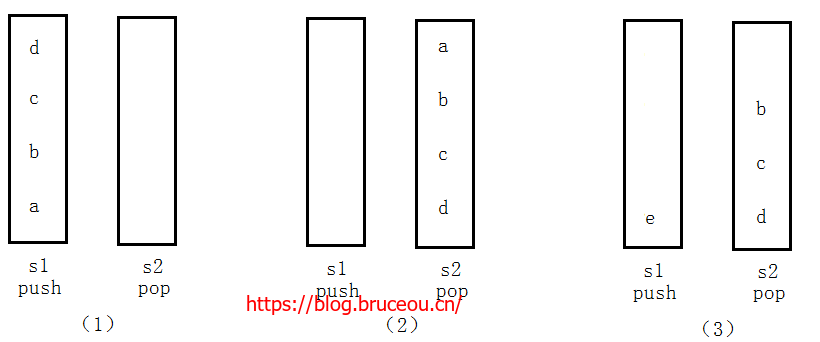### 资源获取方法

1.关注公众号[嵌入式实验楼]
2.在公众号回复关键词[Data Structures and Algorithms]获取资料提取码